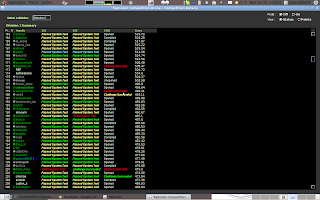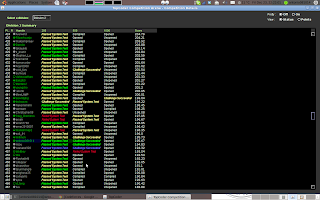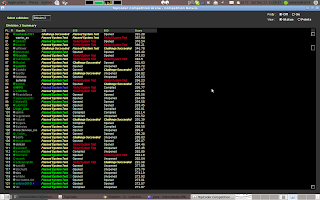Uva12398,

## Problem:

 Problem B: NumPuzz II have recently downloaded an iPhone app called NumPuzz, developed by Marmottajr. I do not read Portugese, but I find this little game quite suitable for our newbies contest. (The app is available for free at the time this problem description is written.)
You are given a 3-by-3 square matrix with integer entries that may range from 0 to 9, and your task is to make all the entries zeros. You may click on a square and have that entry, together with its neighbouring entries, decreased by one. If an entry to be reduced is a zero, it becomes a 9 after the click. Of course, you aim at solving the puzzle with the fewest clicks possible.As a simple example, let us suppose that your initial matrix has two zeros in the last two squares, and 1s everywhere else. If you click on the upper right corner, three of the ones turn into zeros. Now it takes just one more click to solve the puzzle. See the illustration below.A player shows you his sequence of clicks to solve a NumPuzz instance (not necessarily optimal). Write a program that returns the initial configuration.

## Input

Input contains no more than 100 lines. Each line gives a string of length not exceeding 200, describing the sequence of the player's moves. The meanings of the characters are as follows:The solution discussed above is described by "cd" (click first on c, then on d).

## Output

For each test case, print the corresponding matrix in the beginning of the game, using the format shown below.
Notice that the second line in sample input is empty. It means that no clicks are required to solve that puzzle.

```cd

cdifbgah
```

## Sample Output

```Case #1:
1 1 1
1 1 1
1 0 0
Case #2:
0 0 0
0 0 0
0 0 0
Case #3:
3 3 3
3 4 3
3 3 3```

## Source Code:

/**
* UVa 12398 - NumPuzz I
* Solution:
*/

/**
* @author antonio081014
* @since Dec 31, 2011, 3:23:51 PM
*/
public class Main {

public static final int   N  = 3;
public static final int[] dx = { 0, 1, 0, -1 };
public static final int[] dy = { 1, 0, -1, 0 };

public static void main(String[] args) throws Exception {
String strLine;
for (int t = 1; (strLine = br.readLine()) != null; t++) {
int[][] matrix = new int[N][N];
for (int i = strLine.length() - 1; i >= 0; i--) {
int x = (strLine.charAt(i) - 'a') / 3;
int y = (strLine.charAt(i) - 'a') % 3;
matrix[x][y] = (matrix[x][y] + 1) % 10;
for (int j = 0; j < dx.length; j++) {
int xx = x + dx[j];
int yy = y + dy[j];
if (checkBoundary(xx, yy)) {
matrix[xx][yy] = (matrix[xx][yy] + 1) % 10;
}
}
}
print(t, matrix);
}
}

public static void print(int t, int[][] matrix) {
System.out.println("Case #" + Integer.toString(t) + ":");
for (int i = 0; i < N; i++) {
System.out.print(matrix[i]);
for (int j = 1; j < N; j++) {
System.out.print(" ");
System.out.print(matrix[i][j]);
}
System.out.println();
}
}

public static boolean checkBoundary(int x, int y) {
return (x >= 0 && x < N && y >= 0 && y < N);
}
}

UVa12397,

## Problem:

 Problem A: Roman Numerals

We would like to build Roman numerals with matches. As you know, Roman numerals are based on the following seven characters: I, V, X, L, C, D, M. Here we introduce the LUSIVERS font, in which the respective characters look like this:Write a program that counts the number of matches used to build Roman numerals in the LUSIVERS font. This number is exactly the total number of "match heads" in the characters. For instance, to make the number 14 (=XIV), five matches are used.You must follow the "standard modern Roman numerals" (as shown on the Wikipedia page). Expressions like IC or IIII are not allowed.

## Input

Input contains multiple lines, each giving a value of N (1 ≤ N ≤ 3999).

## Output

For each test case, output the number of matches required to build the number N in Roman numerals.

```14
2011
```

```5
11
```

## Source Code:

/*
* UVa 12397, Roman Numerals.
* Solution:
*/

class Main {

private static int[] nums = { 1000, 900, 500, 400, 100, 90, 50, 40, 10, 9,
5, 4, 1          };

public static void main(String[] args) throws Exception {
String strLine;
while ((strLine = br.readLine()) != null) {
int N = Integer.parseInt(strLine);
int count = 0;
for (int i = 0; i < nums.length; i++) {
while (N >= nums[i]) {
N -= nums[i];
count += getMatches(nums[i]);
}
}
System.out.println(count);
}
}

/**
* @return the number of corresponding matches required for the number.
*
* */
public static int getMatches(int number) {
switch (number) {
case 1000:
return 4;
case 900:
return 6;
case 500:
return 3;
case 400:
return 5;
case 100:
return 2;
case 90:
return 4;
case 50:
return 2;
case 40:
return 4;
case 10:
return 2;
case 9:
return 3;
case 5:
return 2;
case 4:
return 3;
case 1:
return 1;
default:
return 1;
}
}

}

## Thursday, December 29, 2011

### SRM528

Level 1 Level 2 Level 3
Div 1 Level 1 Level 2 Level 3
Div 2 Level 1 Level 2 Level 3SRM528

## Tutorials:

### Source Code:

/**
* Dynamic Programming.
*
* Let dp[i][j] represents the number of eels of length exactly 10 she can produce
* with the first i included elements and j cuts.
*
* dp[i][j] = Math.max(dp[i][j], dp[i - 1][j - k] + tmp);
* k is the cuts could be implemented on the i-th.
* dp[i-1][j-k] represents the maximum number could be produced with first i-1 th elements
* and j-k cuts. tmp is the number produced on ith element with k cuts.
*
*/

/**
* @author antonio081014
* @since Dec 28, 2011, 8:24:07 AM
*/
import java.util.Arrays;

public class Cut {
public int getMaximum(int[] eelLengths, int maxCuts) {
int N = eelLengths.length;
int ret = 0;
int[][] dp = new int[N + 1][maxCuts + 1];
Arrays.sort(eelLengths);
for (int i = 1; i <= N; i++) {
int cuts = (int) ((eelLengths[i - 1] - 1) / 10);
for (int j = 0; j <= maxCuts; j++) {
for (int k = 0; k <= Math.min(j, cuts); k++) {
int tmp = 0;
if (k == 0)
tmp = eelLengths[i - 1] == 10 ? 1 : 0;
else if (k == cuts)
tmp = (int) Math.floor(eelLengths[i - 1] / 10);
else
tmp = k;
dp[i][j] = Math.max(dp[i][j], dp[i - 1][j - k] + tmp);
ret = Math.max(ret, dp[i][j]);
}
}
}
return ret;
}
// <%:testing-code%>
}

### Source Code:

/**
* Straight implementation.
*
* Go through bi-directional on the same time.
* When the two characters are both '?', then add the smaller cost of oCost and xCost.
* When the one of two characters is '?', then add the corresponding cost.
* When none of them are '?', check if they are in the same character.
*/

/**
* @author antonio081014
* @since Dec 28, 2011, 8:17:29 AM
*/

public class MinCostPalindrome {
public int getMinimum(String s, int oCost, int xCost) {
int cost = 0;
for (int i = 0, j = s.length() - 1; i < j; i++, j--) {
if (s.charAt(i) == '?' && s.charAt(j) == '?')
cost += 2 * Math.min(oCost, xCost);
else if (s.charAt(i) == '?')
cost += (s.charAt(j) == 'x' ? xCost : oCost);
else if (s.charAt(j) == '?')
cost += (s.charAt(i) == 'x' ? xCost : oCost);
else if (s.charAt(i) != s.charAt(j))
return -1;
}
return cost;
}
// <%:testing-code%>
}

### SRM526.5

Level 1 Level 2 Level 3
Div 1 Level 1 Level 2 Level 3
Div 2 Level 1 Level 2 Level 3SRM526.5

## Tutorials:

### Source Code:

/**
* Method 2: using dynamic programming, which will not work if n is large.
* it will exceed the memory limit.
*/

/**
* @author antonio081014
* @since Dec 23, 2011, 6:07:14 PM
*/

public class MagicCandy {

public int whichOne(int n) {
int step = 0;
while (n > 1) {
step++;
n -= (int) Math.sqrt(n);
}
int e = 1;
for (int i = 0; i < step; i++)
e += (int) Math.sqrt(e + Math.sqrt(e));
return e;
}

public int[] map;
public int[] counts;

public int whichOne2(int n) {

map = new int[n + 1];
counts = new int[n + 1];
int count = 0;
for (int i = 1; i <= n; i++) {
if (isMagic(i)) {
count++;
counts[i] = 1;
}
map[i] = count;
}
int ret = -1;
int mmax = 0;
for (int i = 1; i <= n; i++) {
if (getDepth(i) > mmax) {
mmax = counts[i];
ret = i;
}
}
return ret;
}

public int getDepth(int n) {
if (counts[n] != 0)
return counts[n];
counts[n] = 1 + getDepth(n - map[n]);
return counts[n];
}

public boolean isMagic(int n) {
int x = (int) Math.sqrt(n);
if (n == x * x)
return true;
return false;
}

// <%:testing-code%>
}

### Source Code:

/**
* There exists another easier method based on the observation.
* Any none-one number could be represented by the combination of prime numbers.
*
*/

/**
* @author antonio081014
* @since Dec 23, 2011, 6:02:46 PM
*/

public class MagicStonesStore {
public String ableToDivide(int n) {
for (int i = 1; i <= n; i++) {
if (isPrime(i) && isPrime(2 * n - i))
return "YES";
}
return "NO";
}

public boolean isPrime(int n) {
if (n <= 1)
return false;
if (n == 2)
return true;
if (n % 2 == 0)
return false;
for (int i = 3; i * i <= n; i++)
if (n % i == 0)
return false;
return true;
}

// <%:testing-code%>
}

## Saturday, December 17, 2011

### SRM527:

Level 1 Level 2 Level 3
Div 1 Level 1 Level 2 Level 3
Div 2 Level 1 Level 2 Level 3

## Tutorials:SRM527

### Solution

It's the same with Div2-Level2.

### Solution

Greedy + brute forces.
1st, convert the coins_sum to binary number.
2nd, if the sum is greater than the N-length binary number could represent, use the first coin (or the one with greatest value) to represent the part which is greater than the one could represent.
3rd, count the number of coins used by now.
4th, use the ones with greatest value to increase the number of coins could be used by split it into 2 coins with its half value. This step is implemented by Greedy.

Above all, I used brute forces here.

### Source Code:

/**
* @author antonio081014
* @since Dec 17, 2011, 9:51:30 AM
*/

public class P8XCoinChangeAnother {

public long[] solve(int N, long coins_sum, long coins_count) {
String sum = Long.toBinaryString(coins_sum);
int len = sum.length();
long[] ret = new long[N];
long cnt = 0;
while (len > N) {
cnt = cnt * 2 + (sum.charAt(0) - '0');
sum = sum.substring(1);
len--;
}
long current = 0;
for (int i = 0; i < N; i++) {
// current += sum.charAt(len - 1 - i) - '0';
ret[i] = sum.charAt(len - i - 1) - '0';
}
ret[N - 1] += 2 * cnt;
// current += 2 * cnt;

for (int i = 0; i < N; i++) {
current += ret[i];
System.out.print(ret[i] + " ");
}
System.out.println(current);
int index = N - 1;
while (coins_count > current && index > 0) {
long tmp = coins_count - current;
if (tmp > ret[index]) {
ret[index - 1] += 2 * ret[index];
current += ret[index];
ret[index] = 0;
index--;
} else {
ret[index - 1] += 2 * tmp;
current += tmp;
ret[index] -= tmp;
break;
}
}

if (current != coins_count) {
ret = new long;
}

return ret;
}

// <%:testing-code%>
}

### Solution

This one could be solved by dynamic programming.
The code will be attached later.

### Source Code:

/**
*
* dp[i][j] represents the maximum score with i nodes and j max-degrees.
*
* Then, we add one node with k-degree, we could get:
* dp[i+1][j+k] = Math.max(dp[i+1][j+k], score[k-1] + dp[i][j]);
*
* At last, we only need to return the maximum value with
* N+1 (since N is the number of available degrees, which is nodes number - 1) nodes,
* and 2*N degree.
* */

/**
* @author antonio081014
* @since Dec 29, 2011, 9:54:00 AM
*/

public class P8XGraphBuilder {

public int[][] dp;

public int solve(int[] scores) {
int N = scores.length;
dp = new int[N + 2][2 * N + 2];
for (int i = 0; i < N + 2; i++)
for (int j = 0; j < 2 * N + 2; j++)
dp[i][j] = Integer.MIN_VALUE;
dp = 0;
for (int i = 0; i <= N; i++) {
for (int j = 0; j <= 2 * N; j++) {
if (dp[i][j] == Integer.MIN_VALUE)
continue;
for (int k = 1; k <= N && k + j <= 2 * N; k++) {
dp[i + 1][j + k] = Math.max(dp[i + 1][j + k], scores[k - 1]
+ dp[i][j]);
}
}
}
return dp[N + 1][2 * N];
}
// <%:testing-code%>
}

### Solution

Totally brute force.

### Source Code:

/**
* @author antonio081014
* @since Dec 17, 2011, 9:02:35 AM
*/

public class P8XMatrixTransformation {
public String solve(String[] original, String[] target) {
return counter(original) == counter(target) ? "YES" : "NO";
}

public int counter(String[] matrix) {
int ret = 0;
for (int i = 0; i < matrix.length; i++) {
for (int j = 0; j < matrix[i].length(); j++) {
if (matrix[i].charAt(j) == '1')
ret++;
}
}
return ret;
}

// <%:testing-code%>
}

## Saturday, December 3, 2011

Dec 03, 2011.
Tutorial

Problem: 133A.

### Source Code:

/**
* Implementation.
*/

/**
* @author antonio081014
* @since Dec 3, 2011, 6:48:07 AM
*/
public class A {

/**
* @param args
*/
public static void main(String[] args) throws Exception {
if (strLine.contains("H") || strLine.contains("Q")
|| strLine.contains("9")) {
System.out.println("YES");
} else
System.out.println("NO");
}

}

### SRM525

Level 1 Level 2 Level 3
Div 1 Level 1 Level 2 Level 3
Div 2 Level 1 Level 2 Level 3

## Tutorials:

Rank: 186.

### Solution

The same with Div2-Level1.

### Solution

Just enumerate every possibility could happen.
I saw people used DP counting the number of 'o' in the rectangle to reduce the time consuming.

### Source Code:

/**
* @author antonio081014
* @since Nov 29, 2011, 4:23:01 AM
*/
import java.util.*;
import java.util.regex.*;
import java.text.*;
import java.math.*;
import java.awt.geom.*;

public class DropCoins {

public int getMinimum(String[] board, int K) {
int N = board.length;
int M = board.length();
int ret = Integer.MAX_VALUE;
for (int x = 0; x < N; x++) {
for (int y = 0; y < M; y++) {
for (int xx = x + 1; xx <= N; xx++) {
for (int yy = y + 1; yy <= M; yy++) {
int count = 0;
for (int i = x; i < xx; i++) {
for (int j = y; j < yy; j++) {
if (board[i].charAt(j) == 'o')
count++;
}
}
if (count == K) {
int sum = 2 * Math.min(x, N - xx)
+ Math.max(x, N - xx) + 2
* Math.min(y, M - yy) + Math.max(y, M - yy);
ret = Math.min(ret, sum);
}

}
}
}
}
if (ret == Integer.MAX_VALUE)
return -1;
return ret;
}
// <%:testing-code%>
}

### Solution

Actually, I used the most dummy way to solve this. (DFS)
The easier way would be check if two cell vertical aligned are both Water.
My solution failed because I didn't check the boundary before I go further.

### Source Code:

/**
* @author antonio081014
* @since Nov 29, 2011, 4:02:41 AM
*/
import java.util.*;
import java.util.regex.*;
import java.text.*;
import java.math.*;
import java.awt.geom.*;

public int N;

boolean[][] mark = new boolean[N];
return "YES";
else
return "NO";
}

public boolean search(boolean[][] mark, int x, int y, String[] road) {
if (x == 0 && y == N - 1)
return true;
if (y >= N)
return false;
mark[x][y] = true;
boolean ret = false;
int xx = 1 - x;
if (road[xx].charAt(y) != 'W' && !mark[xx][y])
ret = ret || search(mark, xx, y, road);
if (y + 1 < N && road[x].charAt(y + 1) != 'W' && !mark[x][y + 1])
ret = ret || search(mark, x, y + 1, road);
if (y + 1 < N && road[xx].charAt(y + 1) != 'W' && !mark[xx][y + 1])
ret = ret || search(mark, xx, y + 1, road);
return ret;
}

// <%:testing-code%>
}

## Thursday, November 17, 2011

### SRM524:

Level 1 Level 2 Level 3
Div 1 Level 1 Level 2 Level 3
Div 2 Level 1 Level 2 Level 3
This contest is probably the best score I ever had.
Keep trying, and keep being part of what you like to do.

## Tutorials:

### Solution

The same with Div2-Level2

### Solution

1st, filter out all the available digits left.
2nd, using BFS to compose every possible number, then check if it's the multiple of the given N.
PS: Redefine the comparator for String is another key for this solution. The default comparator compares the two strings character by character, which take the alphabetical order as higher priority, while the length of the string is set as second.

### Source Code:

/**
* 1st, filter out all the available digits.
*
* 2nd, using BFS to compose every possible digits,
* check the number when it's the multiple of the given N;
*/

/**
* @author antonio081014
* @since Nov 17, 2011, 8:16:26 AM
*/
import java.util.ArrayList;
import java.util.Comparator;
import java.util.List;
import java.util.PriorityQueue;
import java.util.Queue;

public class MultiplesWithLimit {
public List<String> nonForbidden;
public boolean[] visited;

public String minMultiples(int N, int[] forbiddenDigits) {
nonForbidden = new ArrayList<String>();
for (int i = 0; i <= 9; i++) {
boolean flag = true;
for (int j = 0; j < forbiddenDigits.length && flag; j++) {
if (i == forbiddenDigits[j])
flag = false;
}
if (flag)
}

visited = new boolean;
Queue<String> queue = new PriorityQueue<String>(10,
new Comparator<String>() {
// overriding the compare method
public int compare(String i, String j) {
if (i.compareTo(j) == 0)
return 0;
if (i.length() < j.length())
return -1;
if (i.length() == j.length() && i.compareTo(j) < 0)
return -1;
return 1;
}
});
while (queue.isEmpty() == false) {
String top = queue.poll();
String tmp;
for (int i = 0; i < nonForbidden.size(); i++) {
tmp = top + nonForbidden.get(i);
if (tmp.startsWith("0"))
continue;
int number = compose(tmp, N);
if (visited[number])
continue;
if (number == 0) {
return getReturn(tmp);
}
visited[number] = true;
}
}
return "IMPOSSIBLE";
}

public String getReturn(String s) {
if (s.length() < 9)
return s;
else
return s.substring(0, 3) + "..." + s.substring(s.length() - 3)
+ "(" + s.length() + " digits)";
}

public int compose(String s, int N) {
int sum = 0;
for (int i = 0; i < s.length(); i++) {
sum = sum * 10 + s.charAt(i) - '0';
sum %= N;
}
return sum;
}

// <%:testing-code%>
}

### Solution

Take the closest non-prime number, which is smaller than n. Greedy Algorithm.

### Source Code:

/**
*
*/

/**
* @author antonio081014
* @since Nov 17, 2011, 8:08:21 AM
*/

public class MagicDiamonds {
public long minimalTransfer(long n) {
long count = 0;
while (n > 0) {
count++;
n -= closestNonePrime(n);
}
return count;
}

public long closestNonePrime(long n) {
for (long x = n; x >= 1; x--) {
if (isPrime(x) == false)
return x;
}
return -1;
}

public boolean isPrime(long n) {
if (n == 2)
return true;
if (n <= 1)
return false;
if (n % 2 == 0)
return false;
for (long i = 3; i * i <= n; i += 2) {
if (n % i == 0)
return false;
}
return true;
}

// <%:testing-code%>
}

### Solution

Iterate every possible combination of a, b, c. Then check if is smaller than what I have already.

### Source Code:

/**
*
*/

/**
* @author antonio081014
* @since Nov 17, 2011, 8:03:13 AM
*/
import java.util.*;
import java.util.regex.*;
import java.text.*;
import java.math.*;
import java.awt.geom.*;

public class ShippingCubes {
public int minimalCost(int N) {
int mmin = Integer.MAX_VALUE;
for (int a = 1; a <= N; a++) {
for (int b = 1; b <= N; b++) {
for (int c = 1; c <= N; c++) {
if (a * b * c == N) {
mmin = Math.min(mmin, a + b + c);
}
}
}
}
return mmin;
}

// <%:testing-code%>
}